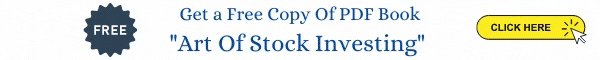## The BETA Of SENSEX Scrips

Beta measures the sensitivity of a scrips Price movement relative to movement in the SENSEX. Statistically Beta is defined as below:

Beta = Covariance [ (SENSEX, Stock) / Variance (SENSEX) ].

Beta is also calculated by regressing stock returns with the index returns. The regression coefficient thus calculated is the Beta value.

Note: Covariance and variance are calculated from the Daily Returns data of the SENSEX and SENSEX scrips

Source: bseindia